# Preprints

1. Refined Cauchy identity for spin Hall-Littlewood symmetric rational functions (2020) • arXiv:2007.10886 [math.CO] • (PDF)
Abstract

Fully inhomogeneous spin Hall-Littlewood symmetric rational functions $\mathsf{F}_\lambda$ arise in the context of $\mathfrak{sl}(2)$ higher spin six vertex models, and are multiparameter deformations of the classical Hall-Littlewood symmetric polynomials. We obtain a refined Cauchy identity expressing a weighted sum of the product of two $\mathsf{F}_\lambda$’s as a determinant. The determinant is of Izergin-Korepin type: it is the partition function of the six vertex model with suitably decorated domain wall boundary conditions. The proof of equality of two partition functions is based on the Yang-Baxter equation.

We rewrite our Izergin-Korepin type determinant in a different form which includes one of the sets of variables in a completely symmetric way. This determinantal identity might be of independent interest, and also allows to directly link the spin Hall-Littlewood rational functions with (the Hall-Littlewood particular case of) the interpolation Macdonald polynomials. In a different direction, a Schur expansion of our Izergin-Korepin type determinant yields a deformation of Schur symmetric polynomials.

In the spin-$\frac12$ specialization, our refined Cauchy identity leads to a summation identity for eigenfunctions of the ASEP (Asymmetric Simple Exclusion Process), a celebrated stochastic interacting particle system in the Kardar-Parisi-Zhang universality class. This produces explicit integral formulas for certain multitime probabilities in ASEP.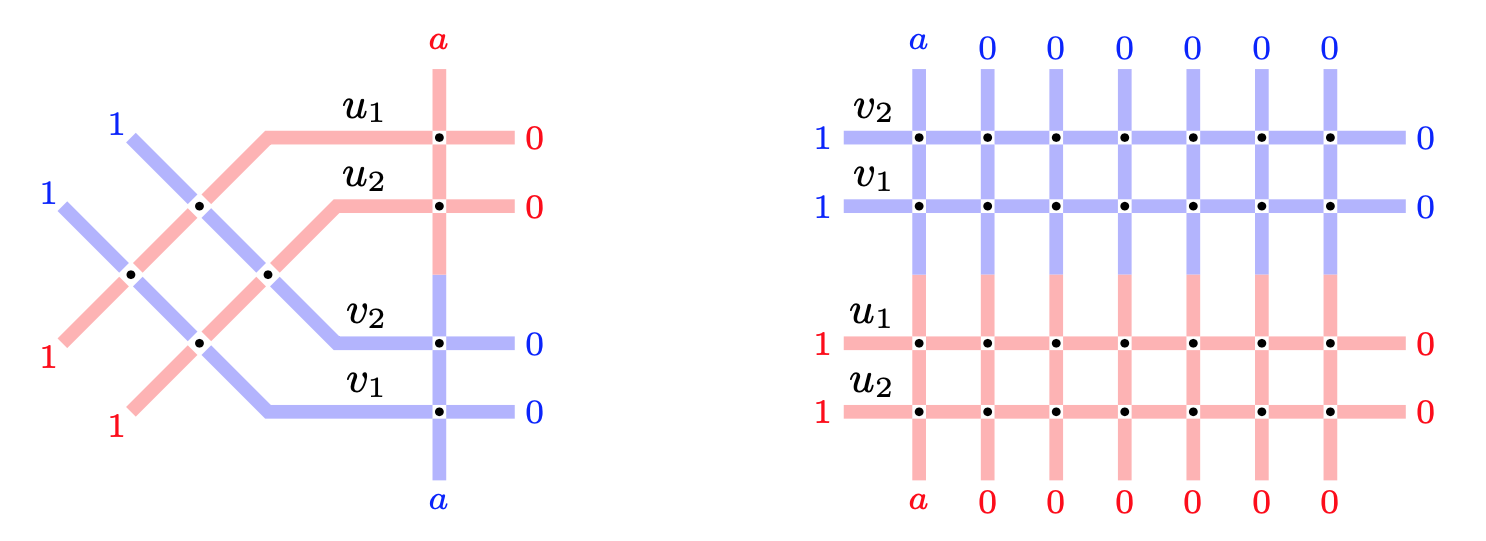2. (with Matteo Mucciconi) Spin q-Whittaker polynomials and deformed quantum Toda (2020) • arXiv:2003.14260 [math.PR] • (PDF) • (TeX)
Abstract

Spin $q$-Whittaker symmetric polynomials labeled by partitions $\lambda$ were recently introduced by Borodin and Wheeler (arXiv:1701.06292) in the context of integrable $\mathfrak{sl}_2$ vertex models. They are a one-parameter deformation of the $t=0$ Macdonald polynomials. We present a new, more convenient modification of spin $q$-Whittaker polynomials and find two Macdonald type $q$-difference operators acting diagonally in these polynomials with eigenvalues, respectively, $q^{-\lambda_1}$ and $q^{\lambda_N}$ (where $\lambda$ is the polynomial’s label). We study probability measures on interlacing arrays based on spin $q$-Whittaker polynomials, and match their observables with known stochastic particle systems such as the $q$-Hahn TASEP.

In a scaling limit as $q\nearrow 1$, spin $q$-Whittaker polynomials turn into a new one-parameter deformation of the $\mathfrak{gl}_n$ Whittaker functions. The rescaled Pieri type rule gives rise to a one-parameter deformation of the quantum Toda Hamiltonian. The deformed Hamiltonian acts diagonally on our new spin Whittaker functions. On the stochastic side, as $q\nearrow 1$ we discover a multilevel extension of the beta polymer model of Barraquand and Corwin (arXiv:1503.04117), and relate it to spin Whittaker functions.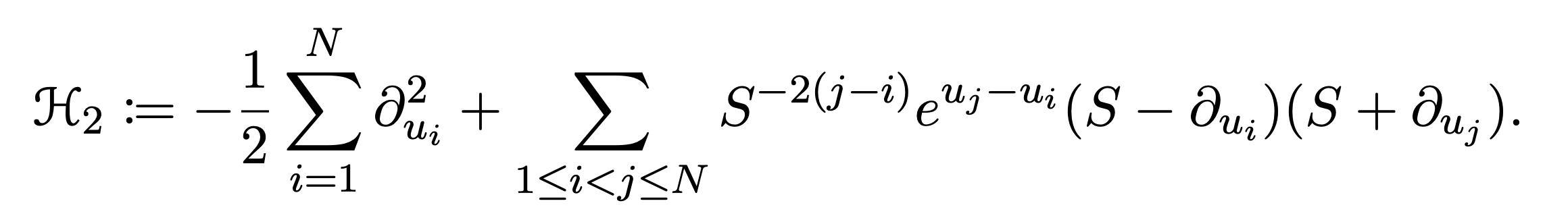# Published or accepted

1. (with Mikhail Tikhonov) Parameter symmetry in perturbed GUE corners process and reflected drifted Brownian motions (2019) • Journal of Statistical Physics, 181 (2020), 1996–2010.arXiv:1912.08671 [math.PR] • (PDF)
Abstract

The perturbed GUE corners ensemble is the joint distribution of eigenvalues of all principal submatrices of a matrix $G+\mathrm{diag}(\mathbf{a})$, where $G$ is the random matrix from the Gaussian Unitary Ensemble (GUE), and $\mathrm{diag}(\mathbf{a})$ is a fixed diagonal matrix. We introduce Markov transitions based on exponential jumps of eigenvalues, and show that their successive application is equivalent in distribution to a deterministic shift of the matrix. This result also leads to a new distributional symmetry for a family of reflected Brownian motions with drifts coming from an arithmetic progression.

The construction we present may be viewed as a random matrix analogue of the recent results of the first author and Axel Saenz.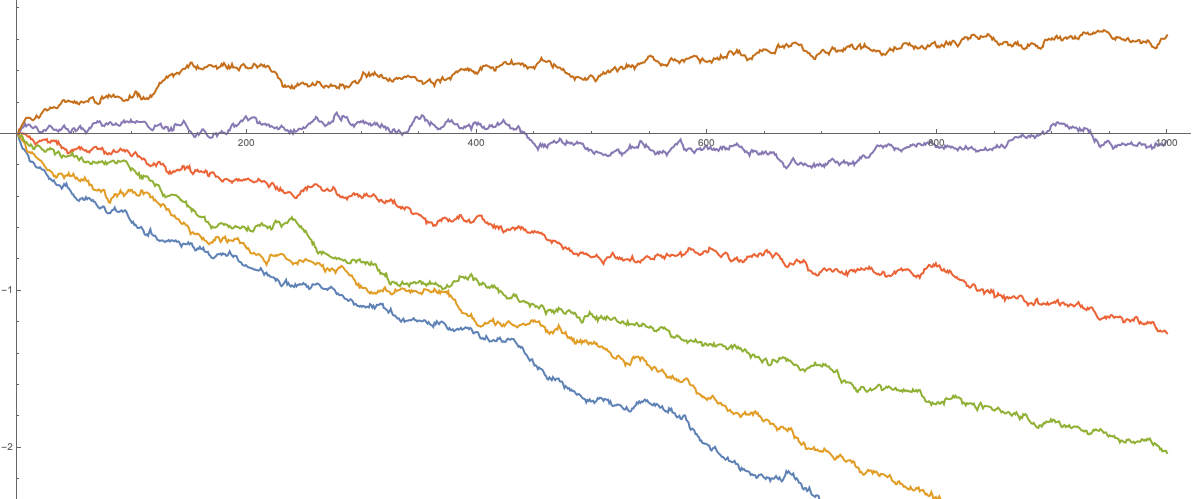2. Parameter permutation symmetry in particle systems and random polymers (2019) • SIGMA 17 (2021), 021, 34 pagesarXiv:1912.06067 [math.PR]
Abstract

Many integrable stochastic particle systems in one space dimension (such as TASEP - Totally Asymmetric Simple Exclusion Process - and its various deformations, with a notable exception of ASEP) remain integrable when we equip each particle $x_i$ with its own jump rate parameter $\nu_i$. It is a consequence of integrability that the distribution of each particle $x_n(t)$ in a system started from the step initial configuration depends on the parameters $\nu_j$, $j\le n$, in a symmetric way. A transposition $\nu_n \leftrightarrow \nu_{n+1}$ of the parameters thus affects only the distribution of $x_n(t)$. For $q$-Hahn TASEP and its degenerations (namely, $q$-TASEP and beta polymer) we realize the transposition $\nu_n \leftrightarrow \nu_{n+1}$ as an explicit Markov swap operator acting on the single particle $x_n(t)$. For beta polymer, the swap operator can be interpreted as a simple modification of the lattice on which the polymer is considered. Our main tools are Markov duality and contour integral formulas for joint moments.

In particular, our constructions lead to a continuous time Markov process $\mathsf{Q}^{(\mathsf{t})}$ preserving the time $\mathsf{t}$ distribution of the $q$-TASEP (with step initial configuration, where $\mathsf{t}\in \mathbb{R}_{>0}$ is fixed). The dual system is a certain transient modification of the stochastic $q$-Boson system. We identify asymptotic survival probabilities of this transient process with $q$-moments of the $q$-TASEP, and use this to show convergence of the process $\mathsf{Q}^{(\mathsf{t})}$ with arbitrary initial data to its stationary distribution.

Setting $q=0$, we recover the results about the usual TASEP established recently in this paper by a different approach based on Gibbs ensembles of interlacing particles in two dimensions.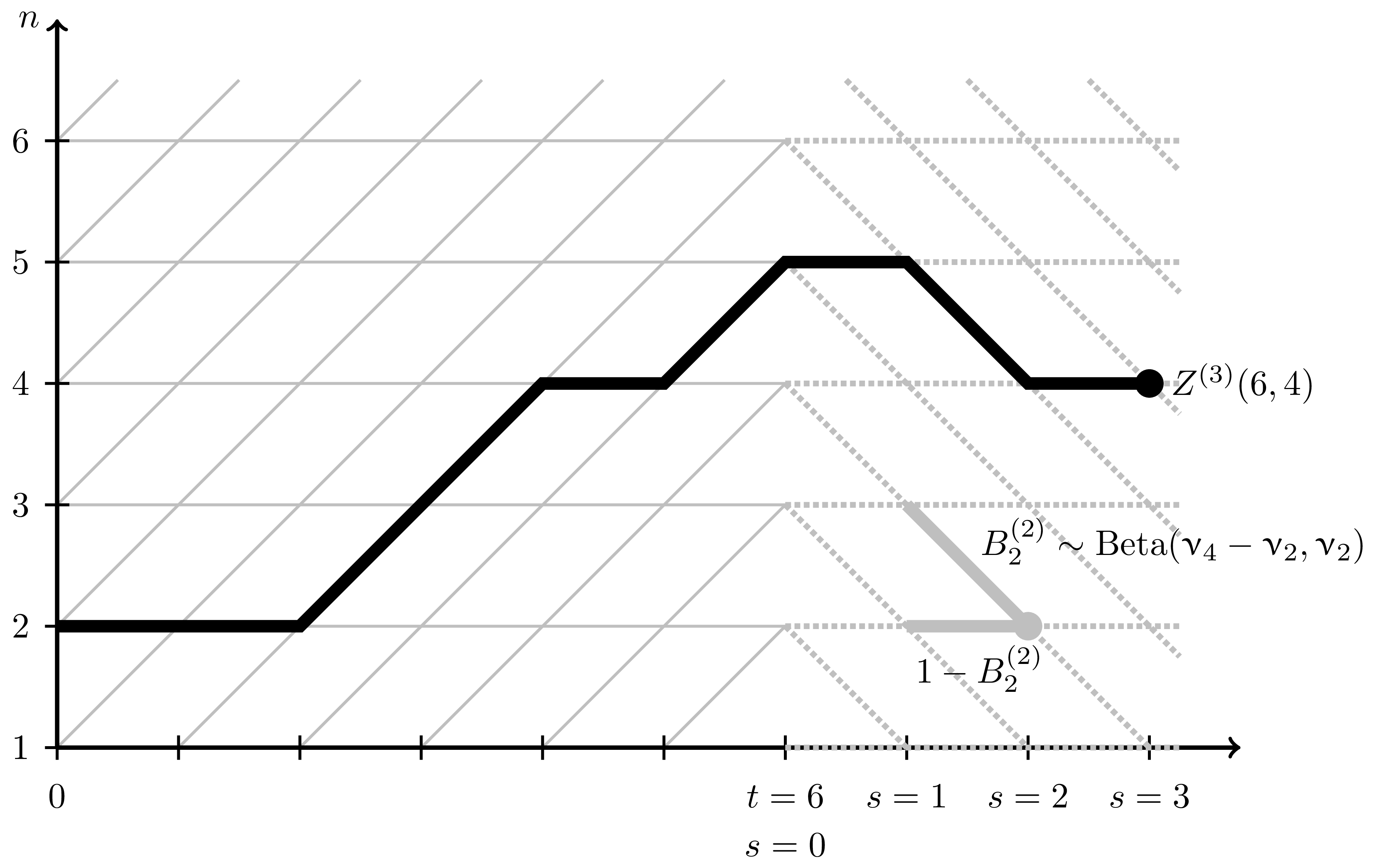3. PushTASEP in inhomogeneous space (2019) • Electronic Journal of Probability, vol. 25 (2020), paper no. 114.arXiv:1910.08994 [math.PR]
Abstract

We consider the PushTASEP (pushing totally asymmetric simple exclusion process, also sometimes called long-range TASEP) with the step initial configuration evolving in an inhomogeneous space. That is, the rate of each particle’s jump depends on the location of this particle. We match the distribution of the height function of this PushTASEP with Schur processes. Using this matching and determinantal structure of Schur processes, we obtain limit shape and fluctuation results which are typical for stochastic particle systems in the Kardar-Parisi-Zhang universality class. PushTASEP is a close relative of the usual TASEP. In inhomogeneous space the former is integrable, while the integrability of the latter is not known.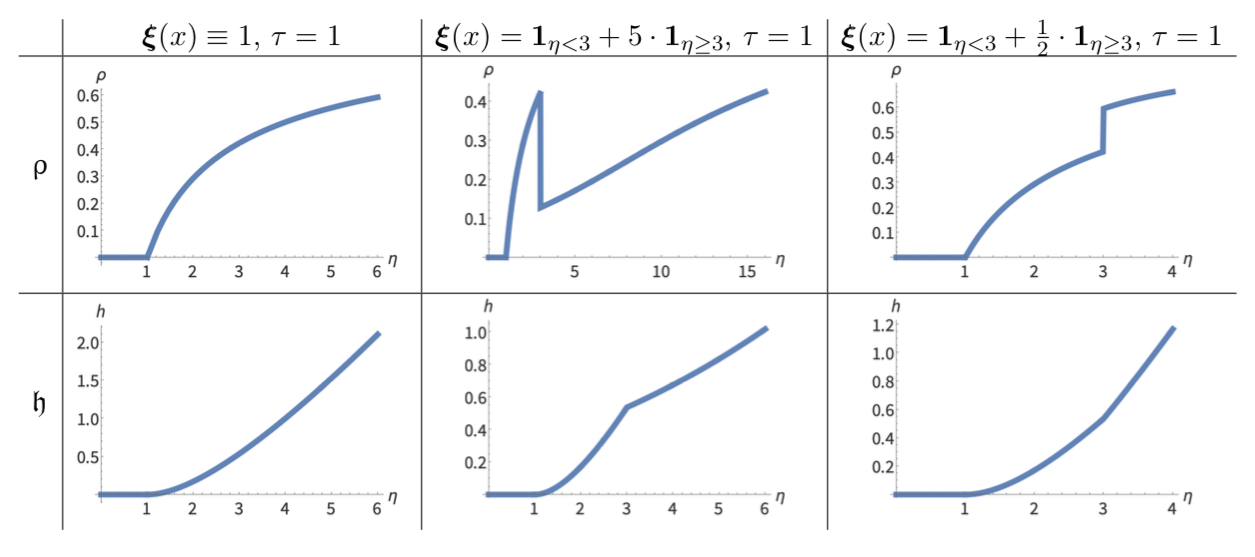4. (with Axel Saenz) Mapping TASEP back in time (2019) • Probability Theory and Related Fields, to appear.arXiv:1907.09155 [math.PR] • (PDF)
Abstract

We obtain a new relation between the distributions $\mu_t$ at different times $t\ge 0$ of the continuous-time TASEP (Totally Asymmetric Simple Exclusion Process) started from the step initial configuration. Namely, we present a continuous-time Markov process with local interactions and particle-dependent rates which maps the TASEP distributions $\mu_t$ backwards in time. Under the backwards process, particles jump to the left, and the dynamics can be viewed as a version of the discrete-space Hammersley process. Combined with the forward TASEP evolution, this leads to an stationary Markov dynamics preserving $\mu_t$ which in turn brings new identities for expectations with respect to $\mu_t$.

The construction of the backwards dynamics is based on Markov maps interchanging parameters of Schur processes, and is motivated by bijectivizations of the Yang-Baxter equation. We also present a number of corollaries, extensions, and open questions arising from our constructions.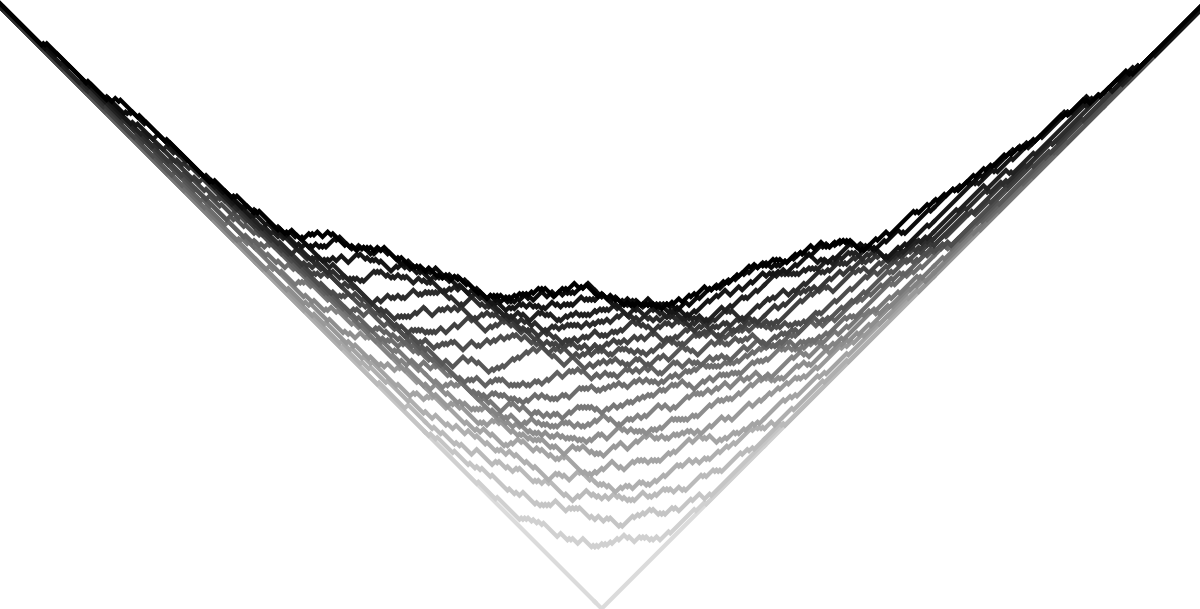5. (with Alexey Bufetov, Matteo Mucciconi) Yang-Baxter random fields and stochastic vertex models (2019) • Advances in Mathematics, to appeararXiv:1905.06815 [math.PR] • (TeX)
Abstract

Bijectivization refines the Yang-Baxter equation into a pair of local Markov moves which randomly update the configuration of the vertex model. Employing this approach, we introduce new Yang-Baxter random fields of Young diagrams based on spin $q$-Whittaker and spin Hall-Littlewood symmetric functions. We match certain scalar Markovian marginals of these fields with (1) the stochastic six vertex model; (2) the stochastic higher spin six vertex model; and (3) a new vertex model with pushing which generalizes the $q$-Hahn PushTASEP introduced recently by Corwin-Matveev-Petrov (2018). Our matchings include models with two-sided stationary initial data, and we obtain Fredholm determinantal expressions for the $q$-Laplace transforms of the height functions of all these models. Moreover, we also discover difference operators acting diagonally on spin $q$-Whittaker or (stable) spin Hall-Littlewood symmetric functions.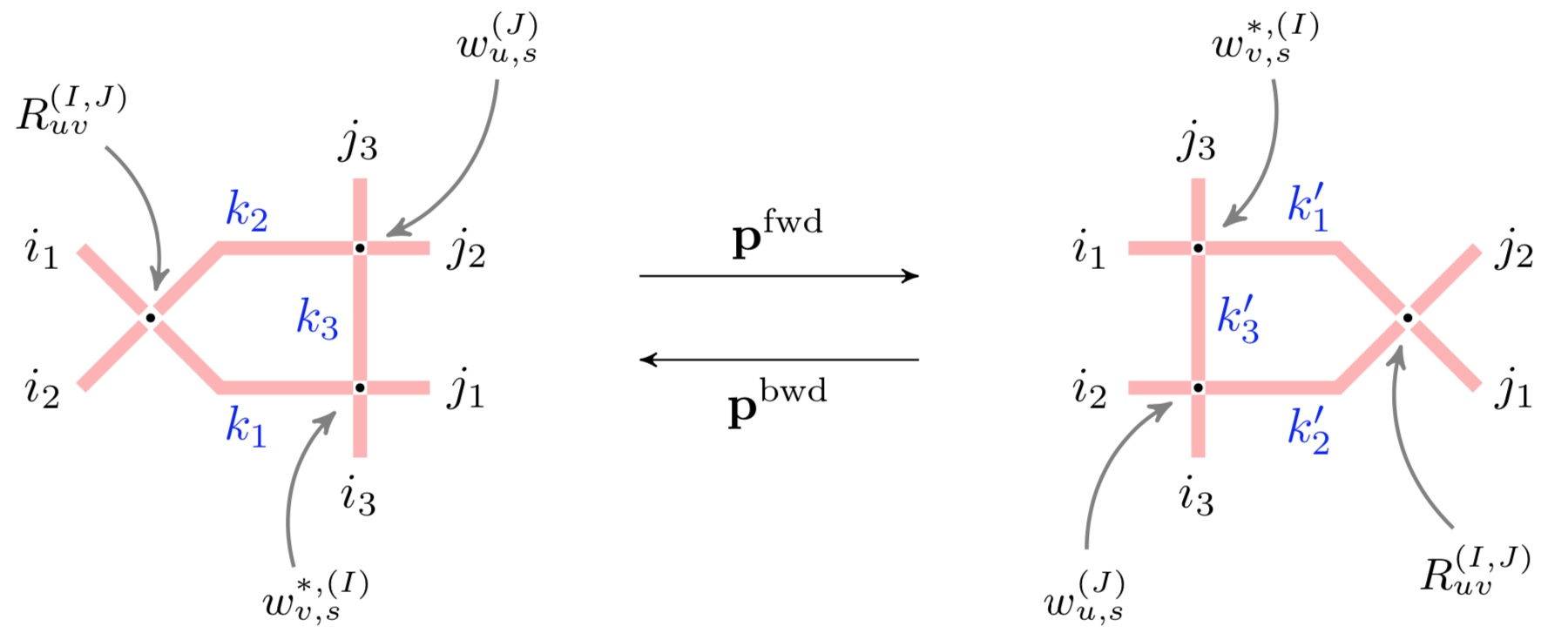6. (with Ivan Corwin, Konstantin Matveev) The q-Hahn PushTASEP (2018) • Intern. Math. Research Notices (2019), rnz106arXiv:1811.06475 [math.PR]
Abstract

We introduce the $q$-Hahn PushTASEP — an integrable stochastic interacting particle system which is a 3-parameter generalization of the PushTASEP, a well-known close relative of the TASEP (Totally Asymmetric Simple Exclusion Process). The transition probabilities in the $q$-Hahn PushTASEP are expressed through the $_4\phi_3$ basic hypergeometric function. Under suitable limits, the $q$-Hahn PushTASEP degenerates to all known integrable (1+1)-dimensional stochastic systems with a pushing mechanism. One can thus view our new system as a pushing counterpart of the $q$-Hahn TASEP introduced by Povolotsky. We establish Markov duality relations and contour integral formulas for the $q$-Hahn PushTASEP. We also take a $q\to1$ limit of our process arriving at a new beta polymer-like model.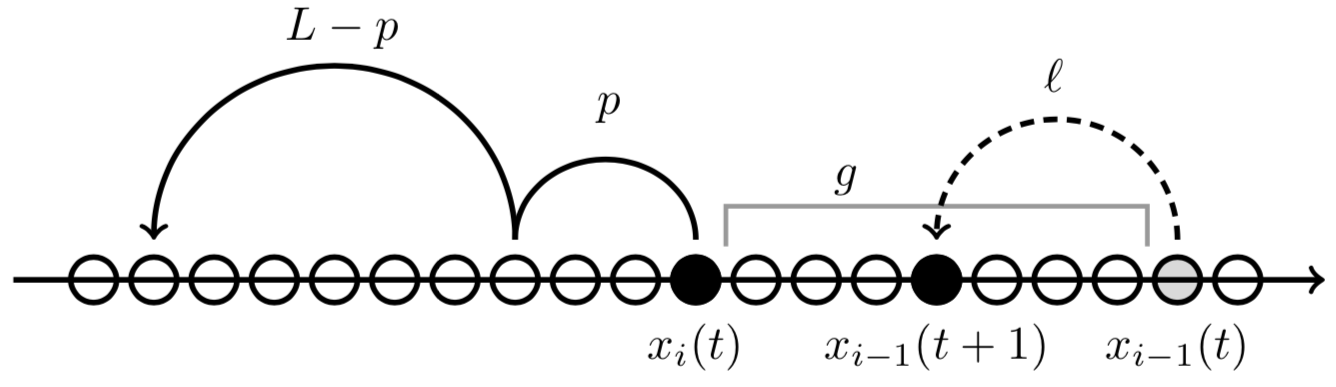7. (with Alisa Knizel, Axel Saenz) Generalizations of TASEP in discrete and continuous inhomogeneous space (2018) • Commun. Math. Phys. 372 (2019), no. 3, pp 797–864.arXiv:1808.09855 [math.PR]
Abstract

We investigate a rich new class of exactly solvable particle systems generalizing the Totally Asymmetric Simple Exclusion Process (TASEP). Our particle systems can be thought of as new exactly solvable examples of tandem queues, directed first- or last passage percolation models, or Robinson-Schensted-Knuth type systems with random input. One of the novel features of the particle systems is the presence of spatial inhomogeneity which can lead to the formation of traffic jams.

For systems with special step-like initial data, we find explicit limit shapes, describe hydrodynamic evolution, and obtain asymptotic fluctuation results which put the systems into the Kardar-Parisi-Zhang universality class. At a critical scaling around a traffic jam in the continuous space TASEP, we observe deformations of the Tracy-Widom distribution and the extended Airy kernel, revealing the finer structure of this novel type of phase transitions.

A homogeneous version of a discrete space system we consider is a one-parameter deformation of the geometric last passage percolation, and we obtain extensions of the limit shape parabola and the corresponding asymptotic fluctuation results.

The exact solvability and asymptotic behavior results are powered by a new nontrivial connection to Schur measures and processes.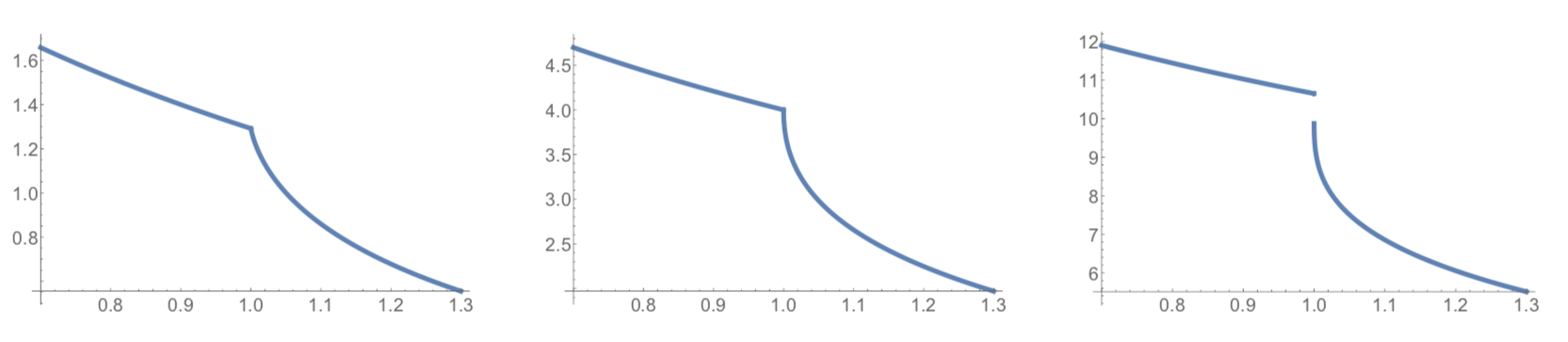8. (with Christian Gromoll, Mark Meckes) Quenched Central Limit Theorem in a Corner Growth Setting (2018) • Electronic Communications in Probability (2018), Vol. 23, paper no. 101, 1-12arXiv:1804.04222 [math.PR]
Abstract

We consider point-to-point directed paths in a random environment on the two-dimensional integer lattice. For a general independent environment under mild assumptions we show that the quenched energy of a typical path satisfies a central limit theorem as the mesh of the lattice goes to zero. Our proofs rely on concentration of measure techniques and some combinatorial bounds on families of paths.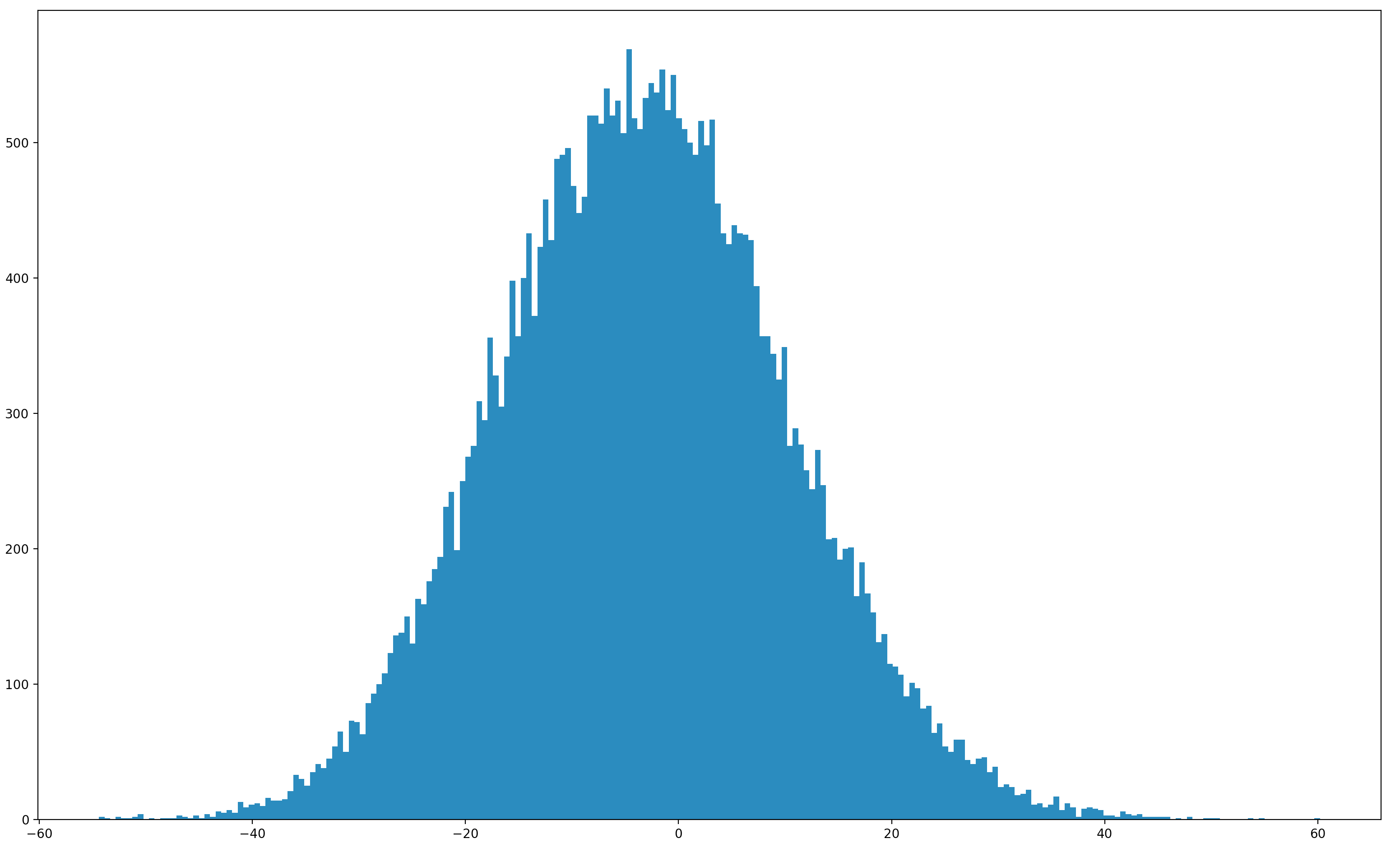9. (with Alexey Bufetov) Yang-Baxter field for spin Hall-Littlewood symmetric functions (2017) • Forum of Mathematics Sigma 7 (2019), e39.arXiv:1712.04584 [math.PR] • (TeX)
Abstract

Employing bijectivisation of summation identities, we introduce local stochastic moves based on the Yang-Baxter equation for $U_q(\widehat{\mathfrak{sl}_2})$. Combining these moves leads to a new object which we call the spin Hall-Littlewood Yang-Baxter field - a probability distribution on two-dimensional arrays of particle configurations on the discrete line. We identify joint distributions along down-right paths in the Yang-Baxter field with spin Hall-Littlewood processes, a generalization of Schur processes. We consider various degenerations of the Yang-Baxter field leading to new dynamic versions of the stochastic six vertex model and of the Asymmetric Simple Exclusion Process.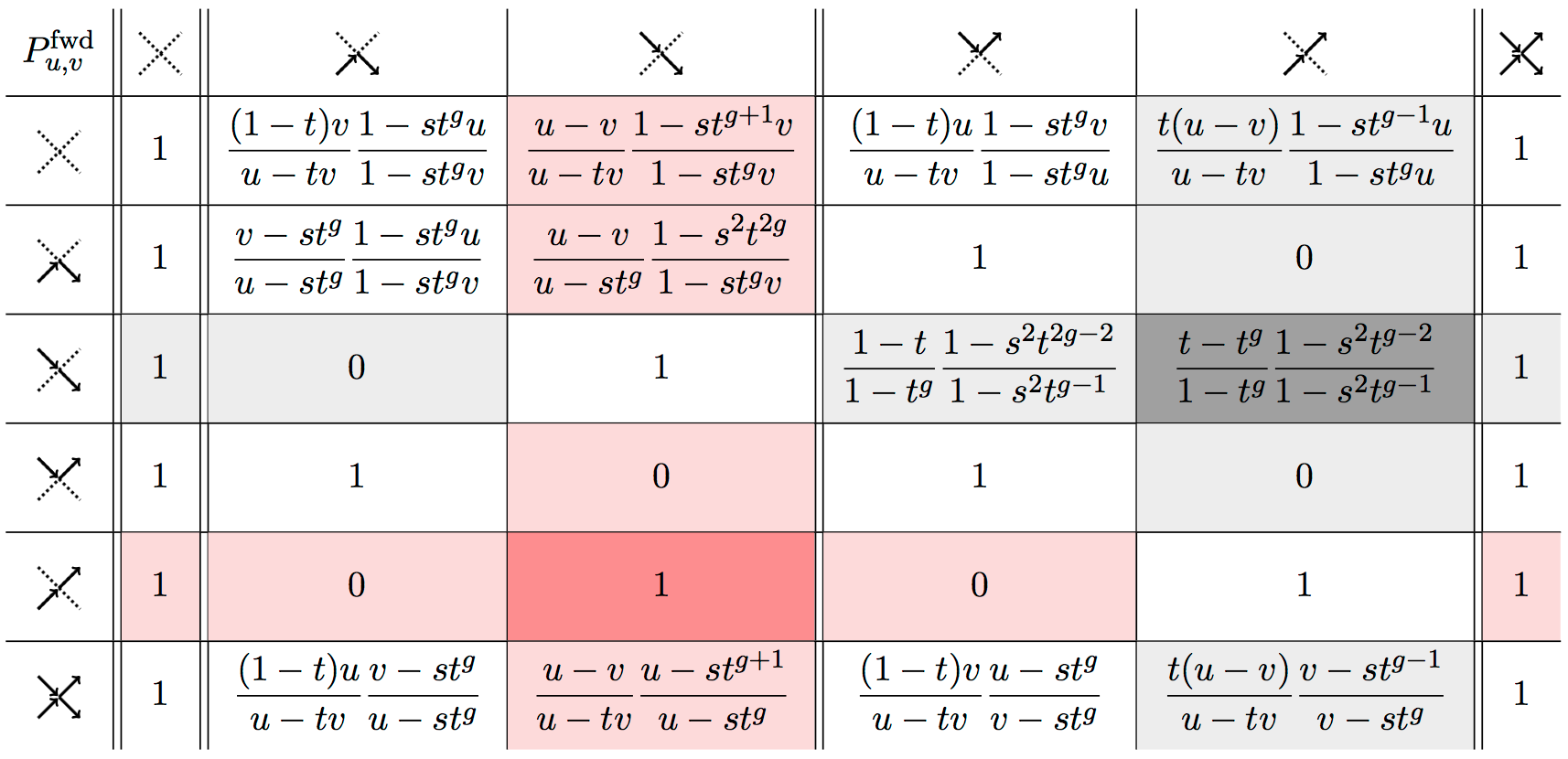10. (with Michael Damron, David Sivakoff) Coarsening model on $\mathbb{Z}^{d}$ with biased zero-energy flips and an exponential large deviation bound for ASEP (2017) • Communications in Mathematical Physics 362 (2018), no. 1, 185–217arXiv:1708.05806 [math.PR]
Abstract

We study the coarsening model (zero-temperature Ising Glauber dynamics) on $\mathbb{Z}^d$ (for $d \geq 2$) with an asymmetric tie-breaking rule. This is a Markov process on the state space ${-1,+1}^{\mathbb{Z}^d}$ of “spin configurations” in which each vertex updates its spin to agree with a majority of its neighbors at the arrival times of a Poisson process. If a vertex has equally many $+1$ and $-1$ neighbors, then it updates its spin value to $+1$ with probability $q \in [0,1]$ and to $-1$ with probability $1-q$. The initial state of this Markov chain is distributed according to a product measure with probability $p$ for a spin to be $+1$.

In this paper, we show that for any given $p>0$, there exist $q$ close enough to 1 such that a.s. every spin has a limit of $+1$. This is of particular interest for small values of $p$, for which it is known that if $q=1/2$, a.s. all spins have a limit of $-1$. For dimension $d=2$, we also obtain near-exponential convergence rates for $q$ sufficiently large, and for general $d$, we obtain stretched exponential rates independent of $d$. Two important ingredients in our proofs are refinements of block arguments of Fontes-Schonmann-Sidoravicius and a novel exponential large deviation bound for the Asymmetric Simple Exclusion Process.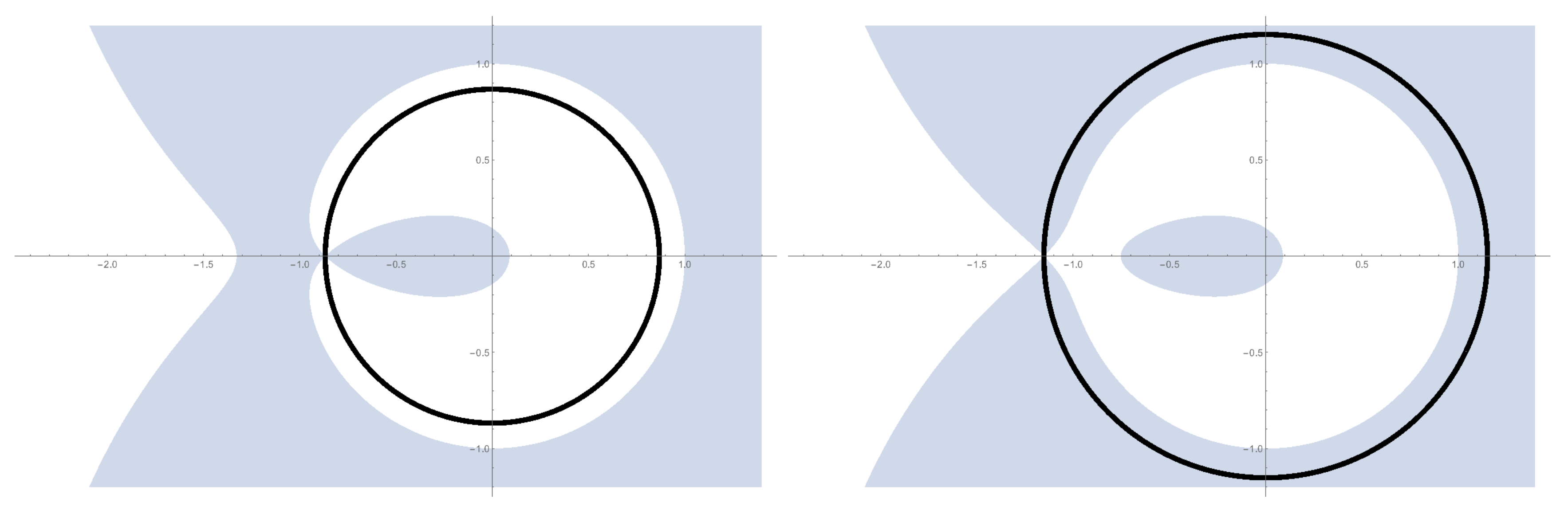11. (with Sevak Mkrtchyan) GUE corners limit of q-distributed lozenge tilings (2017) • Electronic Journal of Probability, Volume 22 (2017), paper no. 101, 24 pp.arXiv:1703.07503 [math.PR]
Abstract

We study asymptotics of $q$-distributed random lozenge tilings of sawtooth domains (equivalently, of random interlacing integer arrays with fixed top row). Under the distribution we consider each tiling is weighted proportionally to $q^{\mathsf{vol}}$, where $\mathsf{vol}$ is the volume under the corresponding 3D stepped surface. We prove the following Interlacing Central Limit Theorem: as $q\rightarrow1$, the domain gets large, and the fixed top row approximates a given nonrandom profile, the vertical lozenges are distributed as the eigenvalues of a GUE random matrix and of its successive principal corners. Our results extend the GUE corners asymptotics for tilings of bounded polygonal domains previously known in the uniform (i.e., $q=1$) case. Even though $q$ goes to $1$, the presence of the $q$-weighting affects non-universal constants in our Central Limit Theorem.12. (with Alexei Borodin) Inhomogeneous exponential jump model (2017) • Probability Theory and Related Fields 172 (2018), 323-385arXiv:1703.03857 [math.PR]
Abstract

We introduce and study the inhomogeneous exponential jump model — an integrable stochastic interacting particle system on the continuous half line evolving in continuous time. An important feature of the system is the presence of arbitrary spatial inhomogeneity on the half line which does not break the integrability. We completely characterize the macroscopic limit shape and asymptotic fluctuations of the height function (= integrated current) in the model. In particular, we explain how the presence of inhomogeneity may lead to macroscopic phase transitions in the limit shape such as shocks or traffic jams. Away from these singularities the asymptotic fluctuations of the height function around its macroscopic limit shape are governed by the GUE Tracy–Widom distribution. A surprising result is that while the limit shape is discontinuous at a traffic jam caused by a macroscopic slowdown in the inhomogeneity, fluctuations on both sides of such a traffic jam still have the GUE Tracy–Widom distribution (but with different non-universal normalizations).

The integrability of the model comes from the fact that it is a degeneration of the inhomogeneous stochastic higher spin six vertex models studied earlier in arXiv:1601.05770 [math.PR].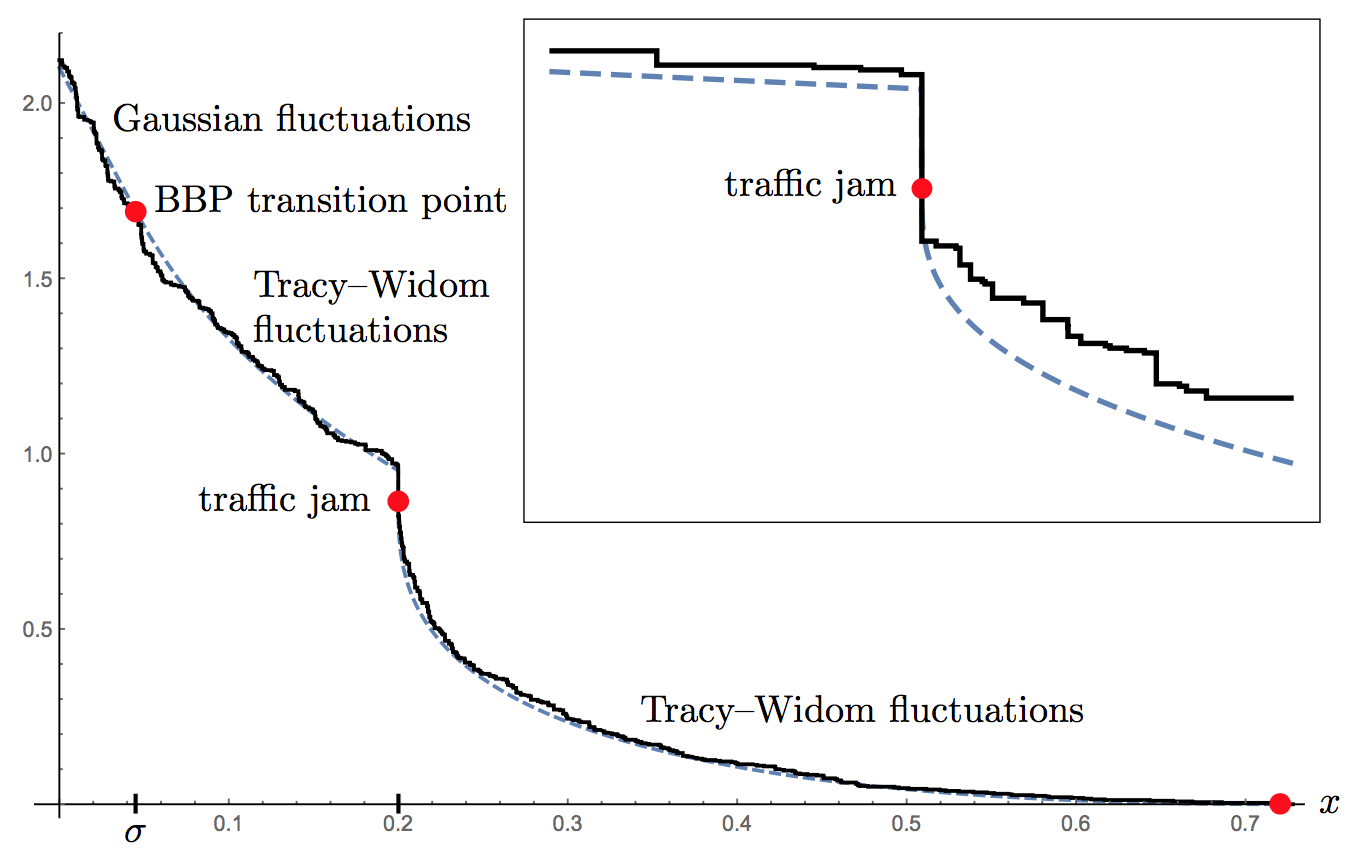13. (with Daniel Orr) Stochastic higher spin six vertex model and q-TASEPs (2016) • Advances in Mathematics 317 (2017), 473-525arXiv:1610.10080 [math.PR]
Abstract

We present two new connections between the inhomogeneous stochastic higher spin six vertex model in a quadrant and integrable stochastic systems from the Macdonald processes hierarchy.

First, we show how Macdonald $q$-difference operators with $t=0$ (an algebraic tool crucial for studying the corresponding Macdonald processes) can be utilized to get $q$-moments of the height function $\mathfrak{h}$ in the higher spin six vertex model first computed in arXiv:1601.05770 [math.PR] using Bethe ansatz. This result in particular implies that for the vertex model with the step Bernoulli boundary condition, the value of $\mathfrak{h}$ at an arbitrary point $(N+1,T)\in\mathbb{Z}_{\ge2}\times\mathbb{Z}_{\ge1}$ has the same distribution as the last component $\lambda_N$ of a random partition under a specific $t=0$ Macdonald measure.

On the other hand, it is known that $\mathbf{x}_N:=\lambda_N-N$ can be identified with the location of the $N$th particle in a certain discrete time $q$-TASEP started from the step initial configuration. The second construction we present is a coupling of this $q$-TASEP and the higher spin six vertex model (with the step Bernoulli boundary condition) along time-like paths providing an independent probabilistic explanation of the equality of $\mathfrak{h}(N+1,T)$ and $\mathbf{x}_N+N$ in distribution. Combined with the identification of averages of observables between the stochastic higher spin six vertex model and Schur measures (which are $t=q$ Macdonald measures) obtained recently in arXiv:1608.01553 [math-ph], this produces GUE Tracy–Widom asymptotics for a discrete time $q$-TASEP with the step initial configuration and special jump parameters.14. (with Vadim Gorin) Universality of local statistics for noncolliding random walks (2016) • Ann. Probab. (2019), Vol. 47, No. 5, 2686-2753.arXiv:1608.03243 [math.PR] • (PDF)
Abstract

We consider the $N$-particle noncolliding Bernoulli random walk — a discrete time Markov process in $\mathbb{Z}^{N}$ obtained from a collection of $N$ independent simple random walks with steps $\in{0,1}$ by conditioning that they never collide. We study the asymptotic behavior of local statistics of this process started from an arbitrary initial configuration on short times $T\ll N$ as $N\to+\infty$. We show that if the particle density of the initial configuration is bounded away from $0$ and $1$ down to scales $\mathsf{D}\ll T$ in a neighborhood of size $\mathsf{Q}\gg T$ of some location $x$ (i.e., $x$ is in the bulk’’), and the initial configuration is balanced in a certain sense, then the space-time local statistics at $x$ are asymptotically governed by the extended discrete sine process (which can be identified with a translation invariant ergodic Gibbs measure on lozenge tilings of the plane). We also establish similar results for certain types of random initial data. Our proofs are based on a detailed analysis of the determinantal correlation kernel for the noncolliding Bernoulli random walk.

The noncolliding Bernoulli random walk is a discrete analogue of the $\boldsymbol{\beta}=2$ Dyson Brownian Motion whose local statistics are universality governed by the continuous sine process. Our results parallel the ones in the continuous case. In addition, we naturally include situations with inhomogeneous local particle density on scale $T$, which nontrivially affects parameters of the limiting extended sine process, and in a particular case leads to a new behavior.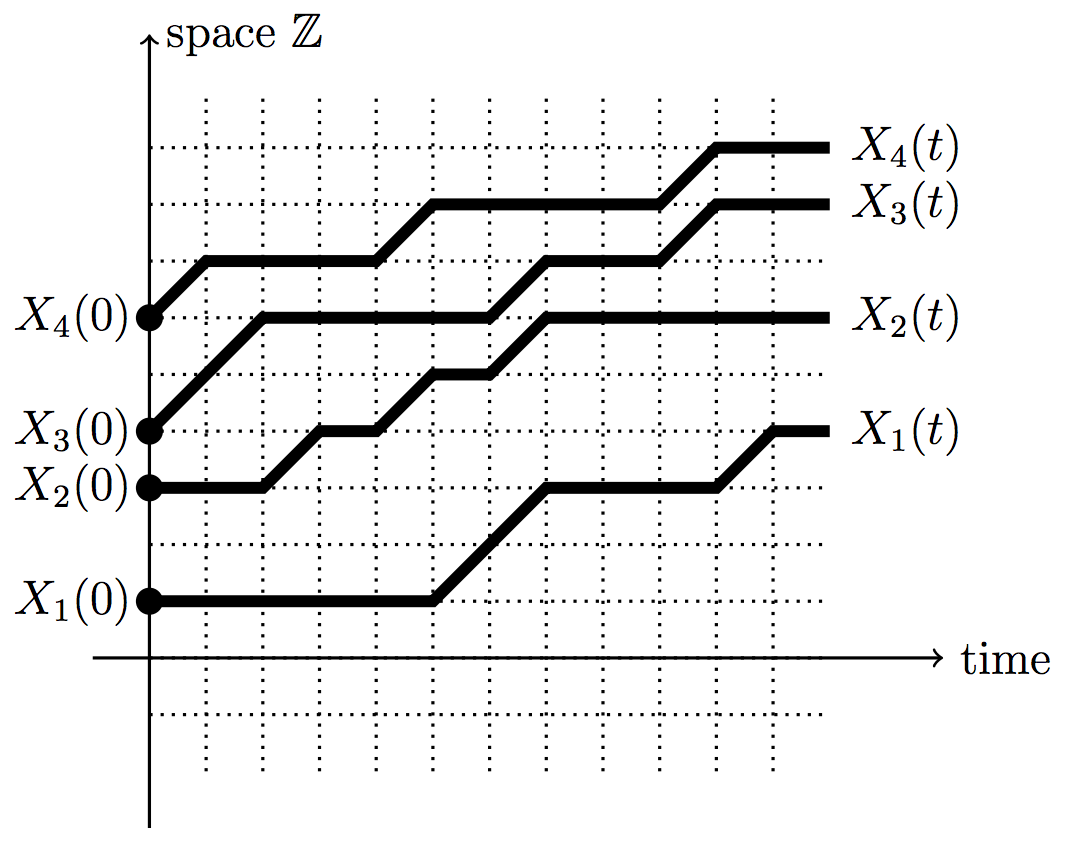15. (with Alexei Borodin) Lectures on Integrable probability: Stochastic vertex models and symmetric functions (2016) • Lecture Notes of the Les Houches Summer School, Volume 104, July 2015arXiv:1605.01349 [math.PR]
Abstract

We consider a homogeneous stochastic higher spin six vertex model in a quadrant. For this model we derive concise integral representations for multi-point q-moments of the height function and for the q-correlation functions. At least in the case of the step initial condition, our formulas degenerate in appropriate limits to many known formulas of such type for integrable probabilistic systems in the (1+1)d KPZ universality class, including the stochastic six vertex model, ASEP, various q-TASEPs, and associated zero range processes.

Our arguments are largely based on properties of a family of symmetric rational functions (introduced in arXiv:1410.0976 [math.CO]) that can be defined as partition functions of the higher spin six vertex model for suitable domains; they generalize classical Hall-Littlewood and Schur polynomials. A key role is played by Cauchy-like summation identities for these functions, which are obtained as a direct corollary of the Yang-Baxter equation for the higher spin six vertex model.

These are lecture notes for a course given by A.B. at the Ecole de Physique des Houches in July of 2015. All the results and proofs presented here generalize to the setting of the fully inhomogeneous higher spin six vertex model, see arXiv:1601.05770 [math.PR] for a detailed exposition of the inhomogeneous case.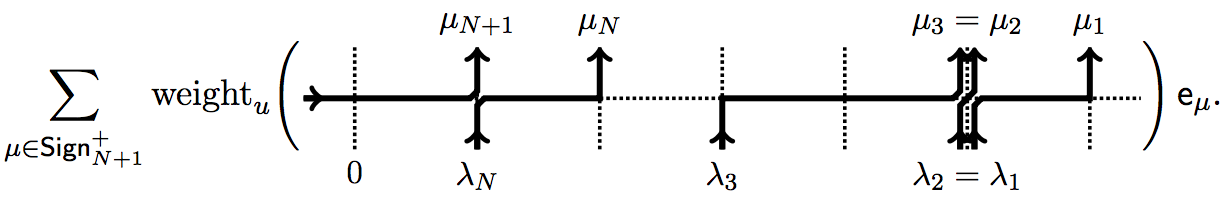16. (with Alexei Borodin) Higher spin six vertex model and symmetric rational functions (2016) • Selecta Math. 24 (2018), no. 2, 751-874arXiv:1601.05770 [math.PR]
Abstract

We consider a fully inhomogeneous stochastic higher spin six vertex model in a quadrant. For this model we derive concise integral representations for multi-point q-moments of the height function and for the q-correlation functions. At least in the case of the step initial condition, our formulas degenerate in appropriate limits to many known formulas of such type for integrable probabilistic systems in the (1+1)d KPZ universality class, including the stochastic six vertex model, ASEP, various q-TASEPs, and associated zero range processes.

Our arguments are largely based on properties of a family of symmetric rational functions which can be defined as partition functions of the inhomogeneous higher spin six vertex model for suitable domains. In the homogeneous case, such functions were previously studied in arXiv:1410.0976 [math.CO]; they also generalize classical Hall-Littlewood and Schur polynomials. A key role is played by Cauchy-like summation identities for these functions, which are obtained as a direct corollary of the Yang-Baxter equation for the higher spin six vertex model.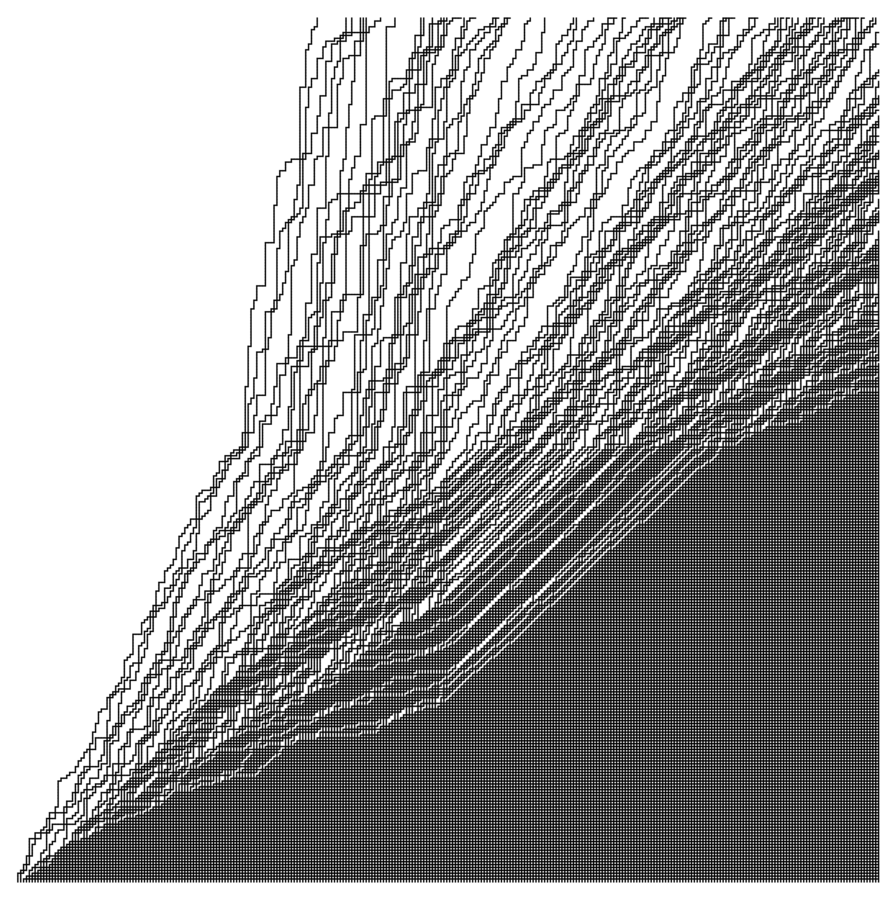17. (with Konstantin Matveev) q-randomized Robinson-Schensted-Knuth correspondences and random polymers (2015) • Annales de l’Institut Henri Poincare D: Combinatorics, Physics and their Interactions 4 (2017), no. 1, 1-123arXiv:1504.00666 [math.PR]
Abstract

We introduce and study $q$-randomized Robinson-Schensted-Knuth (RSK) correspondences which interpolate between the classical ($q=0$) and geometric ($q\to 1$) RSK correspondences (the latter ones are sometimes also called tropical).

For $0< q <1$ our correspondences are randomized, i.e., the result of an insertion is a certain probability distribution on semistandard Young tableaux. Because of this randomness, we use the language of discrete time Markov dynamics on two-dimensional interlacing particle arrays (these arrays are in a natural bijection with semistandard tableaux). Our dynamics act nicely on a certain class of probability measures on arrays, namely, on $q$-Whittaker processes (which are $t=0$ versions of Macdonald processes). We present four Markov dynamics which for $q=0$ reduce to the classical row or column RSK correspondences applied to a random input matrix with independent geometric or Bernoulli entries.

Our new two-dimensional discrete time dynamics generalize and extend several known constructions: (1) The discrete time $q$-TASEPs arise as one-dimensional marginals of our “column” dynamics. In a similar way, our “row” dynamics lead to discrete time $q$-PushTASEPs - new integrable particle systems in the Kardar-Parisi-Zhang universality class. We employ these new one-dimensional discrete time systems to establish a Fredholm determinantal formula for the two-sided continuous time $q$-PushASEP conjectured by Corwin-Petrov (2013). (2) In a certain Poisson-type limit (from discrete to continuous time), our two-dimensional dynamics reduce to the $q$-randomized column and row Robinson-Schensted correspondences introduced by O'Connell-Pei (2012) and Borodin-Petrov (2013), respectively. (3) In a scaling limit as $q\to1$, two of our four dynamics on interlacing arrays turn into the geometric RSK correspondences associated with log-Gamma or strict-weak directed random polymers.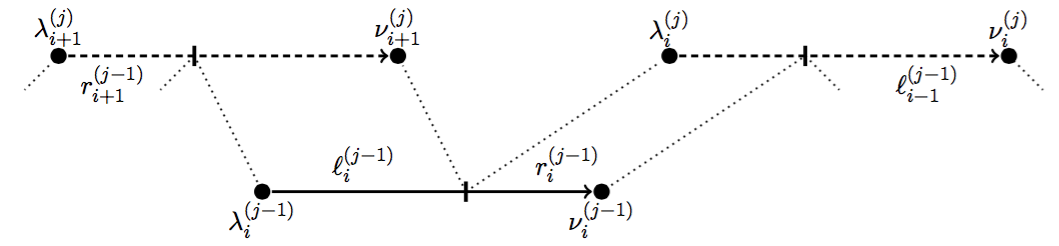18. (with Ivan Corwin) Stochastic higher spin vertex models on the line (2015) • Comm. Math. Phys. 343 (2016), no. 2, 651-700arXiv:1502.07374 [math.PR] • (PDF) • (see also erratum below)
Abstract

We introduce a four-parameter family of interacting particle systems on the line which can be diagonalized explicitly via a complete set of Bethe ansatz eigenfunctions, and which enjoy certain Markov dualities. Using this, for the systems started in step initial data we write down nested contour integral formulas for moments and Fredholm determinant formulas for Laplace-type transforms. Taking various choices or limits of parameters, this family degenerates to many of the known exactly solvable models in the Kardar-Parisi-Zhang universality class, as well as leads to many new examples of such models. In particular, ASEP, the stochastic six-vertex model, $q$-TASEP and various directed polymer models all arise in this manner. Our systems are constructed from stochastic versions of the R-matrix related to the six-vertex model. One of the key tools used here is the fusion of R-matrices and we provide a probabilistic proof of this procedure.

19. (with Alexei Borodin, Ivan Corwin, Tomohiro Sasamoto) Spectral theory for interacting particle systems solvable by coordinate Bethe ansatz (2014) • Comm. Math. Phys. 339 (2015), no. 3, 1167–1245arXiv:1407.8534 [math-ph] • (PDF) • (see also erratum below)
Abstract

We develop spectral theory for the $q$-Hahn stochastic particle system introduced recently by Povolotsky. That is, we establish a Plancherel type isomorphism result which implies completeness and biorthogonality statements for the Bethe ansatz eigenfunctions of the system. Owing to a Markov duality with the $q$-Hahn TASEP (a discrete-time generalization of TASEP with particles’ jump distribution being the orthogonality weight for the classical $q$-Hahn orthogonal polynomials), we write down moment formulas which characterize the fixed time distribution of the $q$-Hahn TASEP with general initial data.

The Bethe ansatz eigenfunctions of the $q$-Hahn system degenerate into eigenfunctions of other (not necessarily stochastic) interacting particle systems solvable by the coordinate Bethe ansatz. This includes the ASEP, the (asymmetric) six-vertex model, and the Heisenberg XXZ spin chain (all models are on the infinite lattice). In this way, each of the latter systems possesses a spectral theory, too. In particular, biorthogonality of the ASEP eigenfunctions which follows from the corresponding $q$-Hahn statement implies symmetrization identities of Tracy and Widom (for ASEP with either step or step Bernoulli initial configuration) as corollaries. Another degeneration takes the $q$-Hahn system to the $q$-Boson particle system (dual to $q$-TASEP) studied in detail in our previous paper (2013).

Thus, at the spectral theory level we unify two discrete-space regularizations of the Kardar-Parisi-Zhang equation / stochastic heat equation, namely, $q$-TASEP and ASEP.

20. (with Alexey Bufetov) Law of Large Numbers for Infinite Random Matrices over a Finite Field (2014) • Selecta Math. 21 (2015), no. 4, 1271–1338arXiv:1402.1772 [math.PR]
Abstract

Asymptotic representation theory of general linear groups $GL(n,q)$ over a finite field leads to studying probability measures $\rho$ on the group $U$ of all infinite uni-uppertriangular matrices over $F_q$, with the condition that $\rho$ is invariant under conjugations by arbitrary infinite matrices. Such probability measures form an infinite-dimensional simplex, and the description of its extreme points (in other words, ergodic measures $\rho$) was conjectured by Kerov in connection with nonnegative specializations of Hall-Littlewood symmetric functions.

Vershik and Kerov also conjectured the following Law of Large Numbers. Consider an $n\times n$ diagonal submatrix of the infinite random matrix drawn from an ergodic measure coming from the Kerov’s conjectural classification. The sizes of Jordan blocks of the submatrix can be interpreted as a (random) partition of $n$, or, equivalently, as a (random) Young diagram $\lambda(n)$ with $n$ boxes. Then, as $n$ goes to infinity, the rows and columns of $\lambda(n)$ have almost sure limiting frequencies corresponding to parameters of this ergodic measure.

Our main result is the proof of this Law of Large Numbers. We achieve it by analyzing a new randomized Robinson-Schensted-Knuth (RSK) insertion algorithm which samples random Young diagrams $\lambda(n)$ coming from ergodic measures. The probability weights of these Young diagrams are expressed in terms of Hall-Littlewood symmetric functions. Our insertion algorithm is a modified and extended version of a recent construction by Borodin and the second author (arXiv:1305.5501). On the other hand, our randomized RSK insertion generalizes a version of the RSK insertion introduced by Vershik and Kerov (1986) in connection with asymptotic representation theory of symmetric groups (which is governed by nonnegative specializations of Schur symmetric functions).

21. (with Alexei Borodin) Integrable probability: From representation theory to Macdonald processes (2013) • Probability Surveys, 11 (2014), 1-58arXiv:1310.8007 [math-ph]
Abstract

These are lecture notes for a mini-course given at the Cornell Probability Summer School in July 2013. Topics include lozenge tilings of polygons and their representation theoretic interpretation, the $(q,t)$-deformation of those leading to the Macdonald processes, nearest neighbor dynamics on Macdonald processes, their limit to semi-discrete Brownian polymers, and large time asymptotic analysis of polymer’s partition function.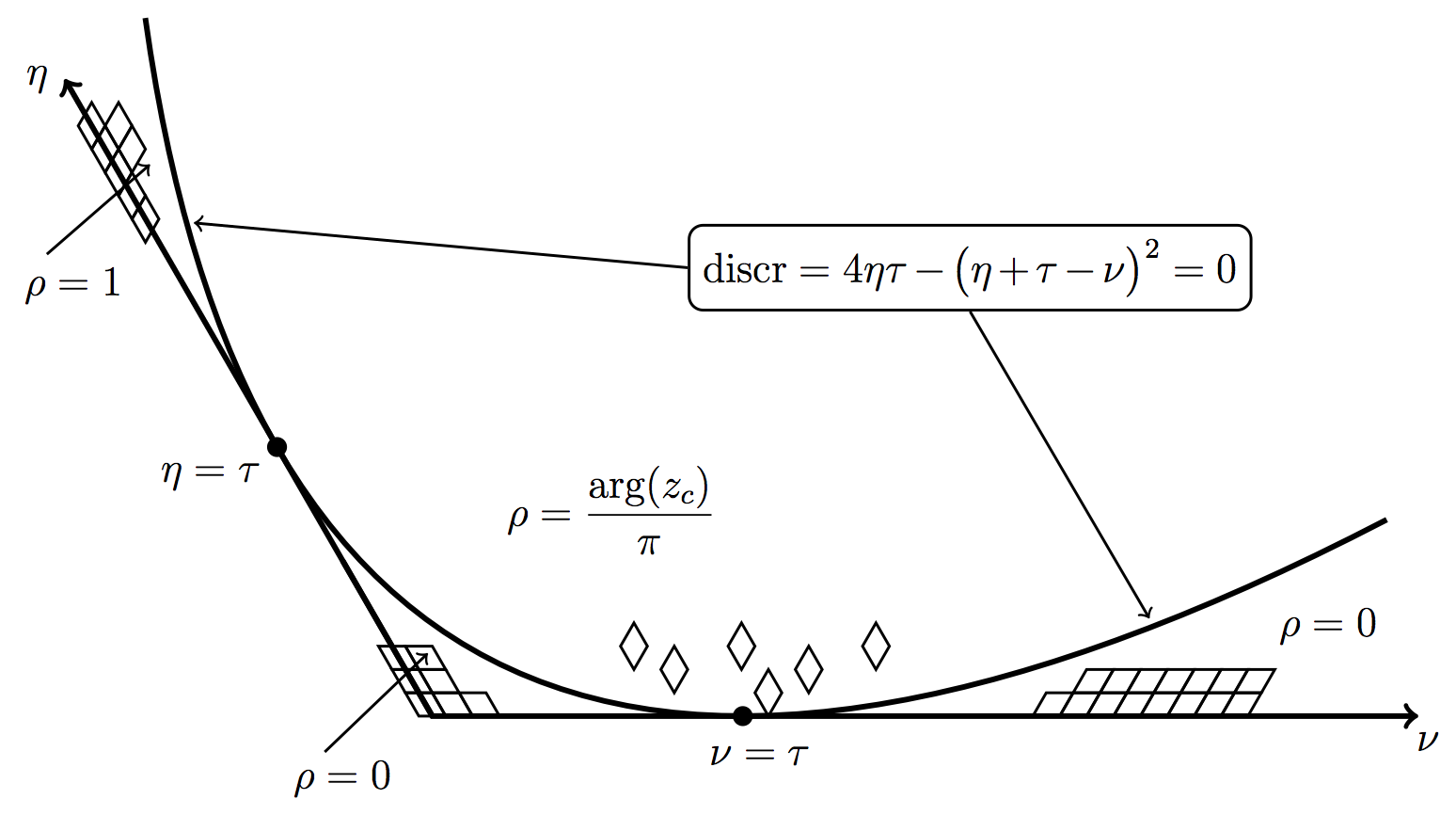22. (with Alexei Borodin, Ivan Corwin, Tomohiro Sasamoto) Spectral theory for the q-Boson particle system (2013) • Compositio Mathematica, 151 (2015), no. 1, 1-67arXiv:1308.3475 [math-ph]
Abstract

We develop spectral theory for the generator of the $q$-Boson (stochastic) particle system. Our central result is a Plancherel type isomorphism theorem for this system. This theorem has various implications. It proves the completeness of the Bethe ansatz for the $q$-Boson generator and consequently enables us to solve the Kolmogorov forward and backward equations for general initial data. Owing to a Markov duality with $q$-TASEP, this leads to moment formulas which characterize the fixed time distribution of $q$-TASEP started from general initial conditions. The theorem also implies the biorthogonality of the left and right eigenfunctions.

We consider limits of our $q$-Boson results to a discrete delta Bose gas considered previously by van Diejen, as well as to another discrete delta Bose gas that describes the evolution of moments of the semi-discrete stochastic heat equation (or equivalently, the O’Connell-Yor semi-discrete directed polymer partition function). A further limit takes us to the delta Bose gas which arises in studying moments of the stochastic heat equation / Kardar-Parisi-Zhang equation.

23. (with Ivan Corwin) The q-PushASEP: A New Integrable Model for Traffic in 1+1 Dimension (2013) • Journal of Statistical Physics, 160 (2015), no. 4, 1005–1026arXiv:1308.3124 [math.PR]
Abstract

We introduce a new interacting (stochastic) particle system $q$-PushASEP which interpolates between the q-TASEP introduced by Borodin and Corwin (see arXiv:1111.4408, and also arXiv:1207.5035; arXiv:1305.2972; arXiv:1212.6716) and the $q$-PushTASEP introduced recently by Borodin and Petrov (arXiv:1305.5501). In the $q$-PushASEP, particles can jump to the left or to the right, and there is a certain partially asymmetric pushing mechanism present. This particle system has a nice interpretation as a model of traffic on a one-lane highway in which cars are able to accelerate or slow down.

Using the quantum many body system approach, we explicitly compute the expectations of a large family of observables for this system in terms of nested contour integrals. We also discuss relevant Fredholm determinantal formulas for the distribution of the location of each particle, and connections of the model with a certain two-sided version of Macdonald processes and with the semi-discrete stochastic heat equation.

24. (with Alexei Borodin) Nearest neighbor Markov dynamics on Macdonald processes (2013) • Advances in Mathematics, 300 (2016), 71-155arXiv:1305.5501 [math.PR]
Abstract

Macdonald processes are certain probability measures on two-dimensional arrays of interlacing particles introduced by Borodin and Corwin (arXiv:1111.4408 [math.PR]). They are defined in terms of nonnegative specializations of the Macdonald symmetric functions and depend on two parameters $(q,t)$, where $0\le q, t < 1$. Our main result is a classification of continuous time, nearest neighbor Markov dynamics on the space of interlacing arrays that act nicely on Macdonald processes.

The classification unites known examples of such dynamics and also yields many new ones. When $t = 0$, one dynamics leads to a new integrable interacting particle system on the one-dimensional lattice, which is a $q$-deformation of the PushTASEP (= long-range TASEP). When $q = t$, the Macdonald processes become the Schur processes of Okounkov and Reshetikhin (arXiv:math/0107056 [math.CO]). In this degeneration, we discover new Robinson–Schensted-type correspondences between words and pairs of Young tableaux that govern some of our dynamics.

25. The Boundary of the Gelfand-Tsetlin Graph: New Proof of Borodin-Olshanski’s Formula, and its q-analogue (2012) • Moscow Mathematical Journal, 14 (2014) no. 1, 121-160arXiv:1208.3443 [math.CO]
Abstract

In the recent paper [arXiv:1109.1412], Borodin and Olshanski have presented a novel proof of the celebrated Edrei-Voiculescu theorem which describes the boundary of the Gelfand-Tsetlin graph as a region in an infinite-dimensional coordinate space. This graph encodes branching of irreducible characters of finite-dimensional unitary groups. Points of the boundary of the Gelfand-Tsetlin graph can be identified with finite indecomposable (= extreme) characters of the infinite-dimensional unitary group. An equivalent description identifies the boundary with the set of doubly infinite totally nonnegative sequences.

A principal ingredient of Borodin-Olshanski’s proof is a new explicit determinantal formula for the number of semi-standard Young tableaux of a given skew shape (or of Gelfand-Tsetlin schemes of trapezoidal shape). We present a simpler and more direct derivation of that formula using the Cauchy-Binet summation involving the inverse Vandermonde matrix. We also obtain a q-generalization of that formula, namely, a new explicit determinantal formula for arbitrary q-specializations of skew Schur polynomials. Its particular case is related to the q-Gelfand-Tsetlin graph and q-Toeplitz matrices introduced and studied by Gorin [arXiv:1011.1769].

26. Asymptotics of uniformly random lozenge tilings of polygons. Gaussian free field (2012) • Annals of Probability, 43 (2014), no. 1, 1–43arXiv:1206.5123 [math.PR]
Abstract

We study large-scale height fluctuations of random stepped surfaces corresponding to uniformly random lozenge tilings of polygons on the triangular lattice. For a class of polygons (which allows arbitrarily large number of sides), we show that these fluctuations are asymptotically governed by a Gaussian free (massless) field. This complements the similar result obtained in Kenyon [Comm. Math. Phys. 281 (2008) 675-709] about tilings of regions without frozen facets of the limit shape. In our asymptotic analysis we use the explicit double contour integral formula for the determinantal correlation kernel of the model obtained previously in arXiv:1202.3901 [math.PR].

27. Asymptotics of Random Lozenge Tilings via Gelfand-Tsetlin Schemes (2012) • Probability Theory and Related Fields, 160 (2014), no. 3, 429–487arXiv:1202.3901 [math.PR]
Abstract

A Gelfand-Tsetlin scheme of depth $N$ is a triangular array with m integers at level $m$, $m=1,\ldots,N$, subject to certain interlacing constraints. We study the ensemble of uniformly random Gelfand-Tsetlin schemes with arbitrary fixed $N$-th row. We obtain an explicit double contour integral expression for the determinantal correlation kernel of this ensemble (and also of its q-deformation).

This provides new tools for asymptotic analysis of uniformly random lozenge tilings of polygons on the triangular lattice; or, equivalently, of random stepped surfaces. We work with a class of polygons which allows arbitrarily large number of sides. We show that the local limit behavior of random tilings (as all dimensions of the polygon grow) is directed by ergodic translation invariant Gibbs measures. The slopes of these measures coincide with the ones of tangent planes to the corresponding limit shapes described by Kenyon and Okounkov in arXiv:math-ph/0507007.

We also prove that at the edge of the limit shape, the asymptotic behavior of random tilings is given by the Airy process.
In particular, our results cover the most investigated case of random boxed plane partitions (when the polygon is a hexagon).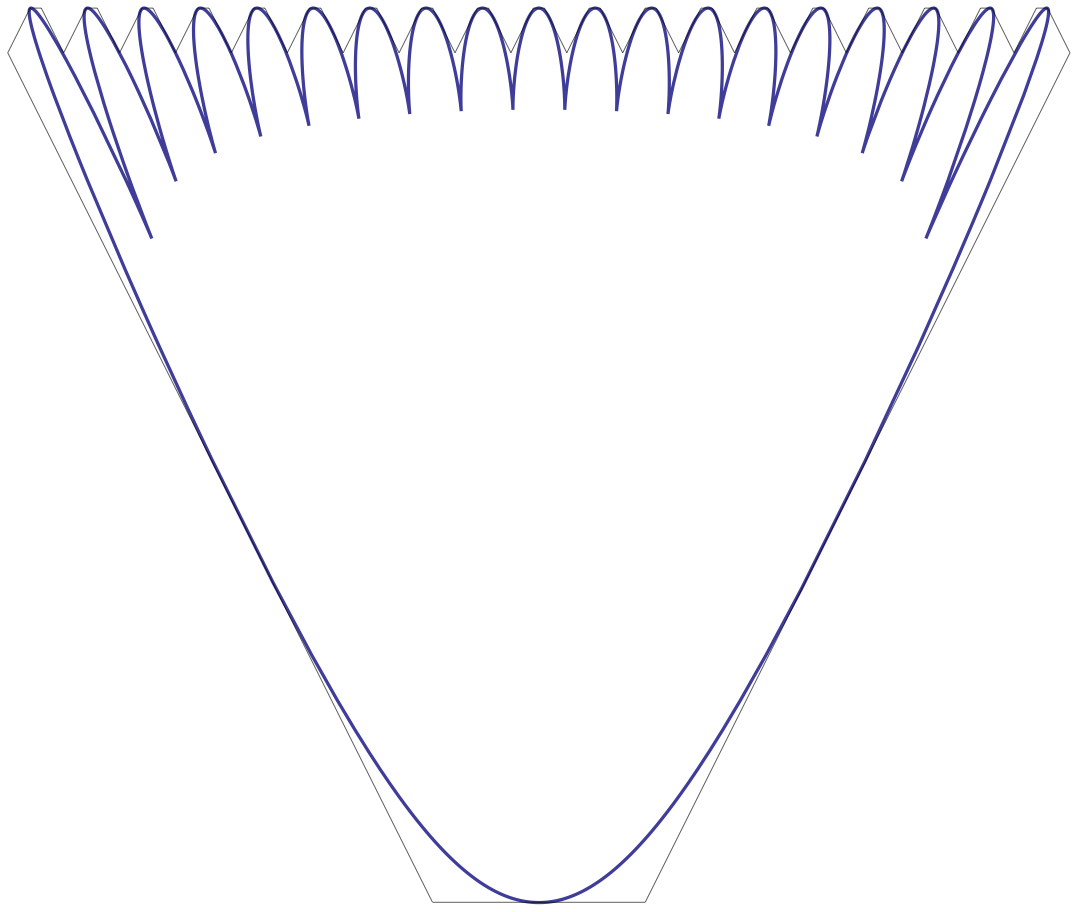28. $\mathfrak{sl}(2)$ Operators and Markov Processes on Branching Graphs (2011) • Journal of Algebraic Combinatorics 38 (2013), no. 3, 663-720arXiv:1111.3399 [math.CO]
Abstract

We present a unified approach to various examples of Markov dynamics on partitions studied by Borodin, Olshanski, Fulman, and the author. Our technique generalizes the Kerov’s operators first appeared in [Okounkov, arXiv:math/0002135], and also stems from the study of duality of graded graphs in [Fomin, 1994].

Our main object is a countable branching graph carrying an $\mathfrak{sl}(2,\mathbb{C})$-module of a special kind. Using this structure, we introduce distinguished probability measures on the floors of the graph, and define two related types of Markov dynamics associated with these measures. We study spectral properties of the dynamics, and our main result is the explicit description of eigenfunctions of the Markov generator of one of the processes.

For the Young graph our approach reconstructs the z-measures on partitions and the associated dynamics studied by Borodin and Olshanski [arXiv:math-ph/0409075, arXiv:0706.1034]. The generator of the dynamics of [arXiv:math-ph/0409075] is diagonal in the basis of the Meixner symmetric functions introduced recently by Olshanski [arXiv:1009.2037, arXiv:1103.5848]. We give new proofs to some of the results of these two papers. Other graphs to which our technique is applicable include the Pascal triangle, the Kingman graph (with the two-parameter Poisson-Dirichlet measures), the Schur graph and the general Young graph with Jack edge multiplicities.

29. On Measures on Partitions Arising in Harmonic Analysis for Linear and Projective Characters of the Infinite Symmetric Group (2011) • Proceedings of the international conference "50 years of IITP"arXiv:1107.0676 [math.CO]
Abstract

The z-measures on partitions originated from the problem of harmonic analysis of linear representations of the infinite symmetric group in the works of Kerov, Olshanski and Vershik (1993, 2004). A similar family corresponding to projective representations was introduced by Borodin (1997). The latter measures live on strict partitions (i.e., partitions with distinct parts), and the z-measures are supported by all partitions. In this note we describe some combinatorial relations between these two families of measures using the well-known doubling of shifted Young diagrams.

30. Pfaffian Stochastic Dynamics of Strict Partitions (2010) • Electronic Journal of Probability 16 (2011), 2246-2295arXiv:1011.3329 [math.PR]
Abstract

We study a family of continuous time Markov jump processes on strict partitions (partitions with distinct parts) preserving the distributions introduced by Borodin (1997) in connection with projective representations of the infinite symmetric group. The one-dimensional distributions of the processes (i.e., the Borodin’s measures) have determinantal structure. We express the dynamical correlation functions of the processes in terms of certain Pfaffians and give explicit formulas for both the static and dynamical correlation kernels using the Gauss hypergeometric function. Moreover, we are able to express our correlation kernels (both static and dynamical) through those of the z-measures on partitions obtained previously by Borodin and Olshanski in a series of papers. The results about the fixed time case were announced in the author’s note arXiv:1002.2714. A part of the present paper contains proofs of those results.

31. Random Strict Partitions and Determinantal Point Processes (2010) • Electronic Communications in Probability 15 (2010), 162-175arXiv:1002.2714 [math.PR]
Abstract

In this note we present new examples of determinantal point processes with infinitely many particles. The particles live on the half-lattice ${1,2,\ldots}$ or on the open half-line $(0,+\infty)$. The main result is the computation of the correlation kernels. They have integrable form and are expressed through the Euler gamma function (the lattice case) and the classical Whittaker functions (the continuous case). Our processes are obtained via a limit transition from a model of random strict partitions introduced by Borodin (1997) in connection with the problem of harmonic analysis for projective characters of the infinite symmetric group.

32. Random Walks on Strict Partitions (2009) • Journal of Mathematical Sciences 168 (2010), no. 3, 437-463arXiv:0904.1823 [math.PR]
Abstract

We consider a certain sequence of random walks. The state space of the n-th random walk is the set of all strict partitions of n (that is, partitions without equal parts). We prove that, as n goes to infinity, these random walks converge to a continuous-time Markov process. The state space of this process is the infinite-dimensional simplex consisting of all nonincreasing infinite sequences of nonnegative numbers with sum less than or equal to one. The main result about the limit process is the expression of its the pre-generator as a formal second order differential operator in a polynomial algebra. Of separate interest is the generalization of Kerov interlacing coordinates to the case of shifted Young diagrams.

33. Limit Behavior of Certain Random Walks on Strict Partitions (2009) • Russian Mathematical Surveys 64 (2009), no. 6, 1139-1141
34. A Two-parameter Family of Infinite-dimensional Diffusions in the Kingman Simplex (2007) • Functional Analysis and Its Applications 43 (2009), no. 4, 279-296arXiv:0708.1930 [math.PR]
Abstract

The aim of the paper is to introduce a two-parameter family of infinite-dimensional diffusion processes $X(\alpha,\theta)$ related to Pitman’s two-parameter Poisson-Dirichlet distributions $PD(\alpha,\theta)$. The diffusions $X(\alpha,\theta)$ are obtained in a scaling limit transition from certain finite Markov chains on partitions of natural numbers. The state space of $X(\alpha,\theta)$ is an infinite-dimensional simplex called the Kingman simplex. In the special case when parameter $\alpha$ vanishes, our finite Markov chains are similar to Moran-type model in population genetics, and our diffusion processes reduce to the infinitely-many-neutral-alleles diffusion model studied by Ethier and Kurtz (1981).

Our main results extend those of Ethier and Kurtz to the two-parameter case and are as follows: The Poisson-Dirichlet distribution $PD(\alpha,\theta)$ is a unique stationary distribution for the corresponding process $X(\alpha,\theta)$; the process is ergodic and reversible; the spectrum of its generator is explicitly described. The general two-parameter case seems to fall outside the setting of models of population genetics, and our approach differs in some aspects from that of Ethier and Kurtz. We also consider the case of degenerate series of parameters $\alpha$ and $\theta$ and conclude that the diffusions in finite-dimensional simplexes studied by Ethier and Kurtz (1981) arise as a special case of our two-parameter family of diffusions.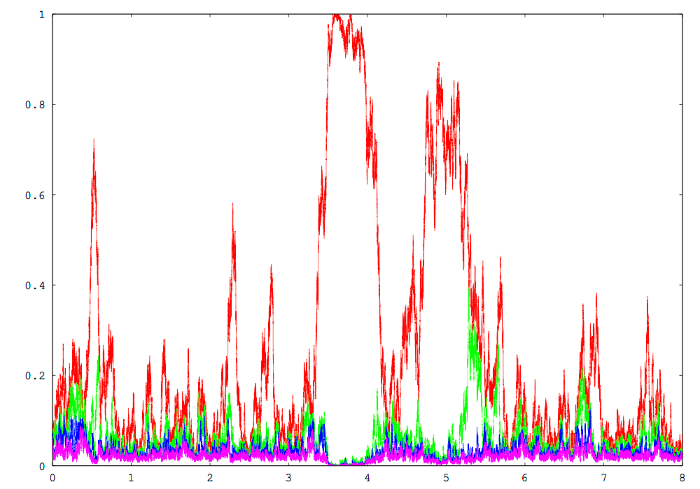35. Asymptotic Behavior of a Certain Collection of Particles on a Line Under Synchronization (2006) • Proceedings of the XXVIII Conference of Young Scientists of Department of Mechanics and Mathematics of the Lomonosov Moscow State University (2006), 152-156, in Russian • • (PDF)

# Other works

1. Sihan Li, Andrew Mecca, Jeewoo Kim, Giusy Caprara, Elizabeth Wagner, Ting-Ting Du, Leonid Petrov, Wenhao Xu, Runjia Cui, Ivan Rebustini, Bechara Kachar, Anthony Peng, and Jung-Bum Shin, Myosin-VIIa is expressed in multiple isoforms and essential for tensioning the hair cell mechanotransduction complex. Nature Communications, 11, Article number: 2066 (2020).

# Errata

1. (with Ivan Corwin) Erratum to "Stochastic higher spin vertex models on the line" (2019) • (PDF)
2. (with Alexei Borodin, Ivan Corwin, Tomohiro Sasamoto) Erratum to "Spectral theory for interacting particle systems solvable by coordinate Bethe ansatz" (2018) • (PDF)

# Slides of some talks

1. Radnom polymers and symmetric functions (2020) • PDF (12.2 MB)
Description

Surveys integrable random polymers (based on gamma / inverse gamma or beta distributed weights), and explains their connection to symmetric functions. Based on a joint work with Matteo Mucciconi.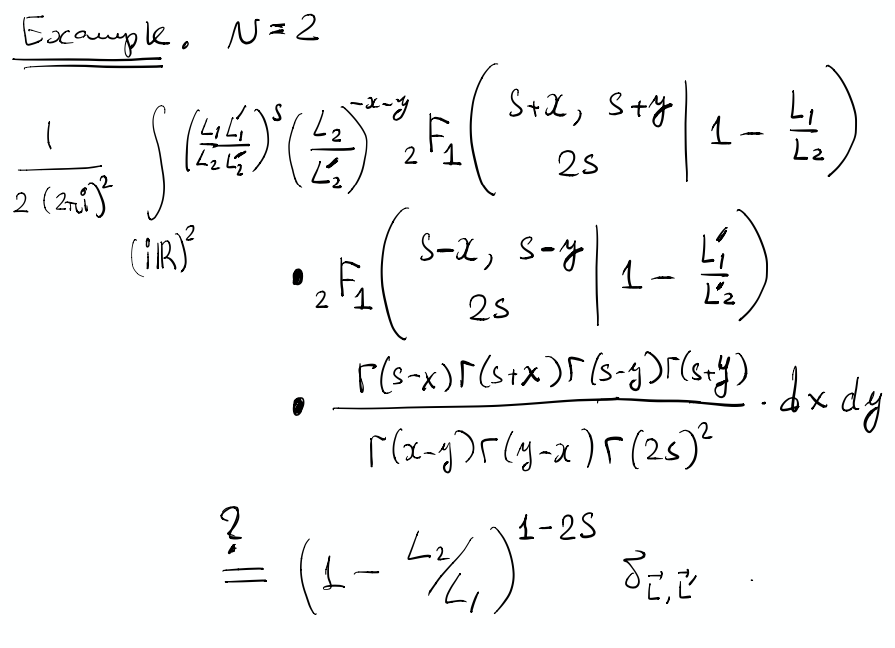2. Spin deformation of q-Whittaker polynomials and Whittaker functions (2020) • PDF (33.6 MB)
Description

Spin $q$-Whittaker symmetric polynomials labeled by partitions $\lambda$ were recently introduced by Borodin and Wheeler (arXiv:1701.06292) in the context of integrable $\mathfrak{sl}_2$ vertex models. They are a one-parameter deformation of the $t=0$ Macdonald polynomials. We present a new, more convenient modification of spin $q$-Whittaker polynomials and find two Macdonald type $q$-difference operators acting diagonally in these polynomials with eigenvalues, respectively, $q^{-\lambda_1}$ and $q^{\lambda_N}$ (where $\lambda$ is the polynomial’s label). We study probability measures on interlacing arrays based on spin $q$-Whittaker polynomials, and match their observables with known stochastic particle systems such as the $q$-Hahn TASEP.

In a scaling limit as $q\nearrow 1$, spin $q$-Whittaker polynomials turn into a new one-parameter deformation of the $\mathfrak{gl}_n$ Whittaker functions. The rescaled Pieri type rule gives rise to a one-parameter deformation of the quantum Toda Hamiltonian. The deformed Hamiltonian acts diagonally on our new spin Whittaker functions. On the stochastic side, as $q\nearrow 1$ we discover a multilevel extension of the beta polymer model of Barraquand and Corwin (arXiv:1503.04117), and relate it to spin Whittaker functions.

Based on a joint work with Matteo Mucciconi and earlier works with Alexey Bufetov and both of them.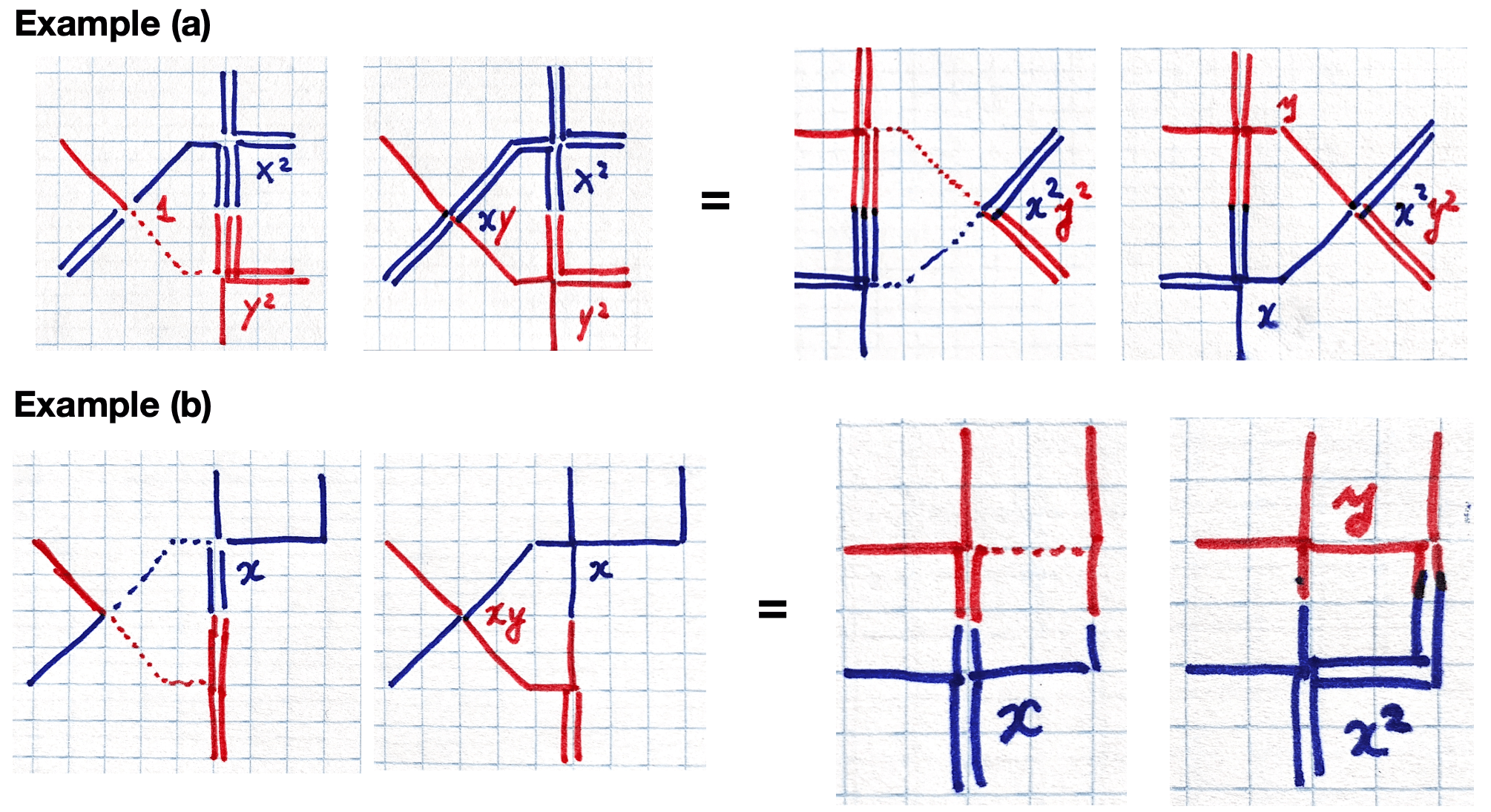3. Mapping TASEP back in time (2019) • PDF (13.5 MB)
Description

We obtain a new relation between the distributions $\mu_t$ at different times $t ≥ 0$ of the continuous-time TASEP (Totally Asymmetric Simple Exclusion Process) started from the step initial configuration. Namely, we present a continuous-time Markov process with local interactions and particle-dependent rates which maps the TASEP distributions μt backwards in time. Under the backwards process, particles jump to the left, and the dynamics can be viewed as a version of the discrete-space Hammersley process. Combined with the forward TASEP evolution, this leads to a stationary Markov dynamics preserving $\mu_t$ which in turn brings new identities for expectations with respect to $\mu_t$. Based on a joint work with Axel Saenz.

Note that the original keynote presentation contained videos which are not included in the PDF download.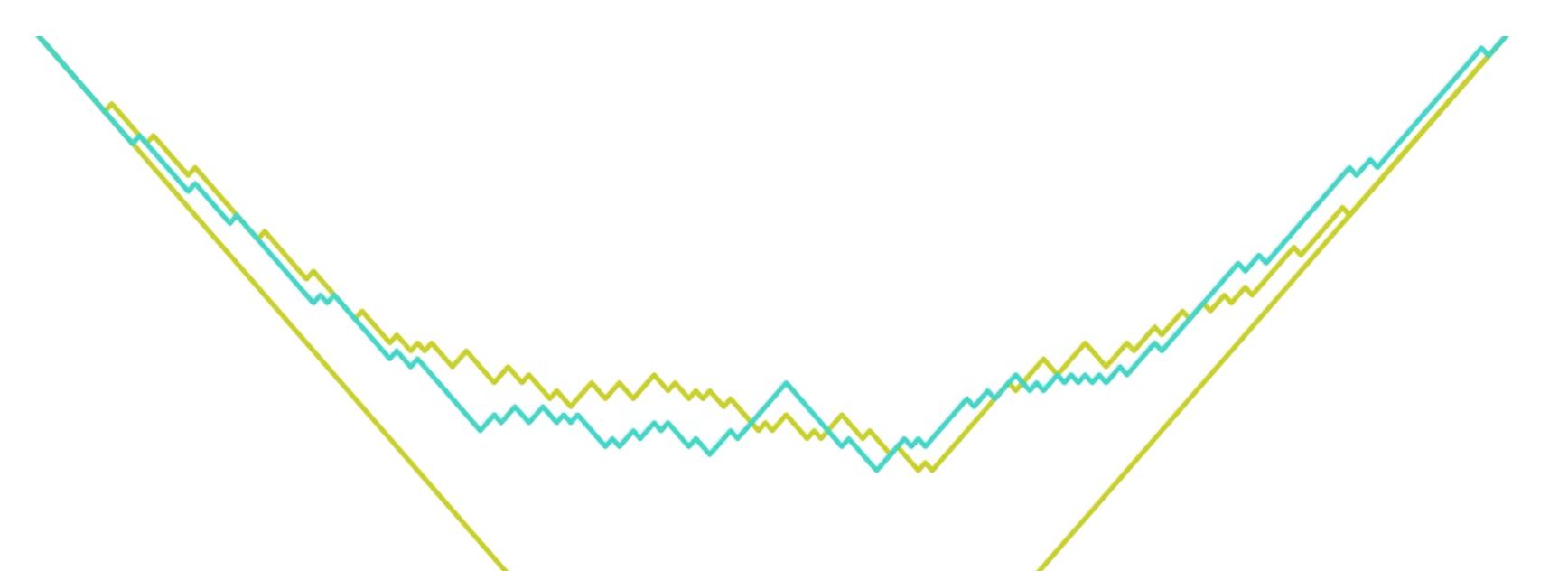4. From infinite random matrices over finite fields to square ice (2019) • PDF (32 MB)
Description

Asymptotic representation theory of symmetric groups is a rich and beautiful subject with deep connections with probability, mathematical physics, and algebraic combinatorics. A one-parameter deformation of this theory related to infinite random matrices over a finite field leads to a randomization of the classical Robinson-Schensted correspondence between words and Young tableaux. Exploring such randomizations we find unexpected applications to six vertex (square ice) type models and traffic systems on a 1-dimensional lattice.5. Gibbs measures, arctic curves, and random interfaces (2018) • PDF (35 MB)
Description

This talk outlines connections between 2-dimensional Gibbs measures with a height function and particle systems in the Kardar-Parisi-Zhang universality class.6. Nonequilibrium particle systems in inhomogeneous space (2018) • PDF (5 MB)
Description

This talk describes results on continuous space TASEP in inhomogeneous space, based on papers with Borodin and with with Knizel and Saenz, and on some work in progress.

7. Spectral theory for interacting particle systems (2014) • PDF (1 MB)
Description

This talk describes results on spectral theory for q-Hahn zero-range process, ASEP, six-vertex model, and q-TASEP. Based on  and .

8. Integrable Probability: Random Polymers, Random Tilings, and Interacting Particle Systems (2013) • PDF (6 MB)
Description

One of the versions of my “job talk” in Fall 2013 describing many facets of integrable probability. It is supposed to be accessible. The talk is based on  and on the previous two talks. More details can also be found in arXiv:1106.1596 [math.PR] by Corwin and arXiv:1212.3351 [math.PR] by Borodin and Gorin.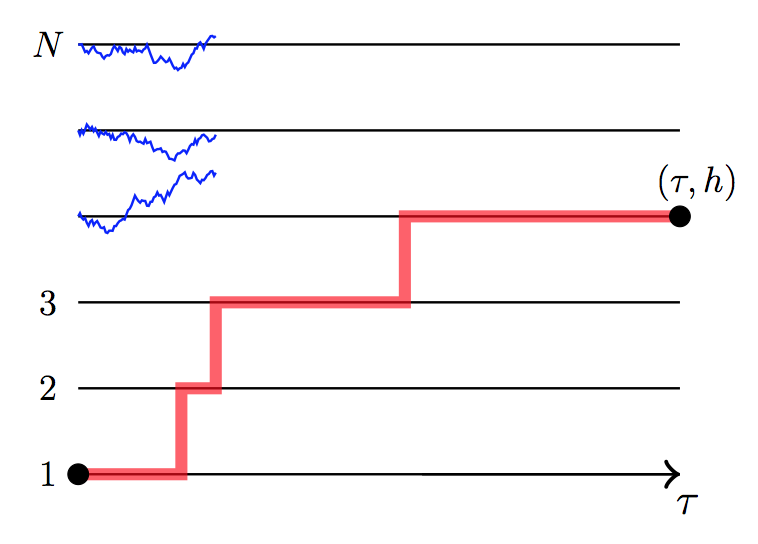9. Markov Dynamics on Interlacing Arrays (2013) • PDF (7 MB)
Description

The talk is devoted to the construction of Markov dynamics on interlacing arrays which act nicely on the Macdonald measures. In a particular case one gets $q$-deformed Robinson-Schensted insertion algorithms. The talk is based on , , see also , .

10. Random 3D surfaces and their asymptotic behavior (2012) • PDF (6 MB)
Description

The talk describes the results of , , and  on asymptotic behavior of random lozenge tilings via determinantal structure and double contour integral formulas for the correlation kernel.

11. $\mathfrak{sl}(2)$ Operators and Markov Dynamics on Branching Graphs (2011) • PDF (1.5 MB)
Description

The talk is based on  and describes $\mathfrak{sl}(2,\mathbb{C})$ structures behind Markov jump processes on the Young and related branching graphs

12. Infinite-dimensional Diffusions Related to the Two-parameter Poisson-Dirichlet Distributions (2010) • PDF (1 MB)
Description

The talk describes population genetics perspective behind infinite-dimensional diffusions preserving the two-parameter Poisson–Dirichlet distributions and related models. It is based on .

13. Infinite-Dimensional Diffusion Processes Approximated by Finite Markov Chains on Partitions (2009) • PDF (2 MB)
Description

The talk describes algebraic/combinatorial perspective behind infinite-dimensional diffusions preserving the two-parameter Poisson–Dirichlet distributions and related models. It is based on , see also ,